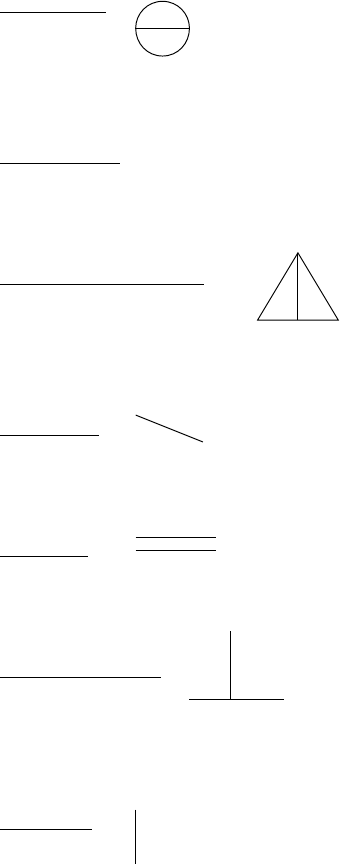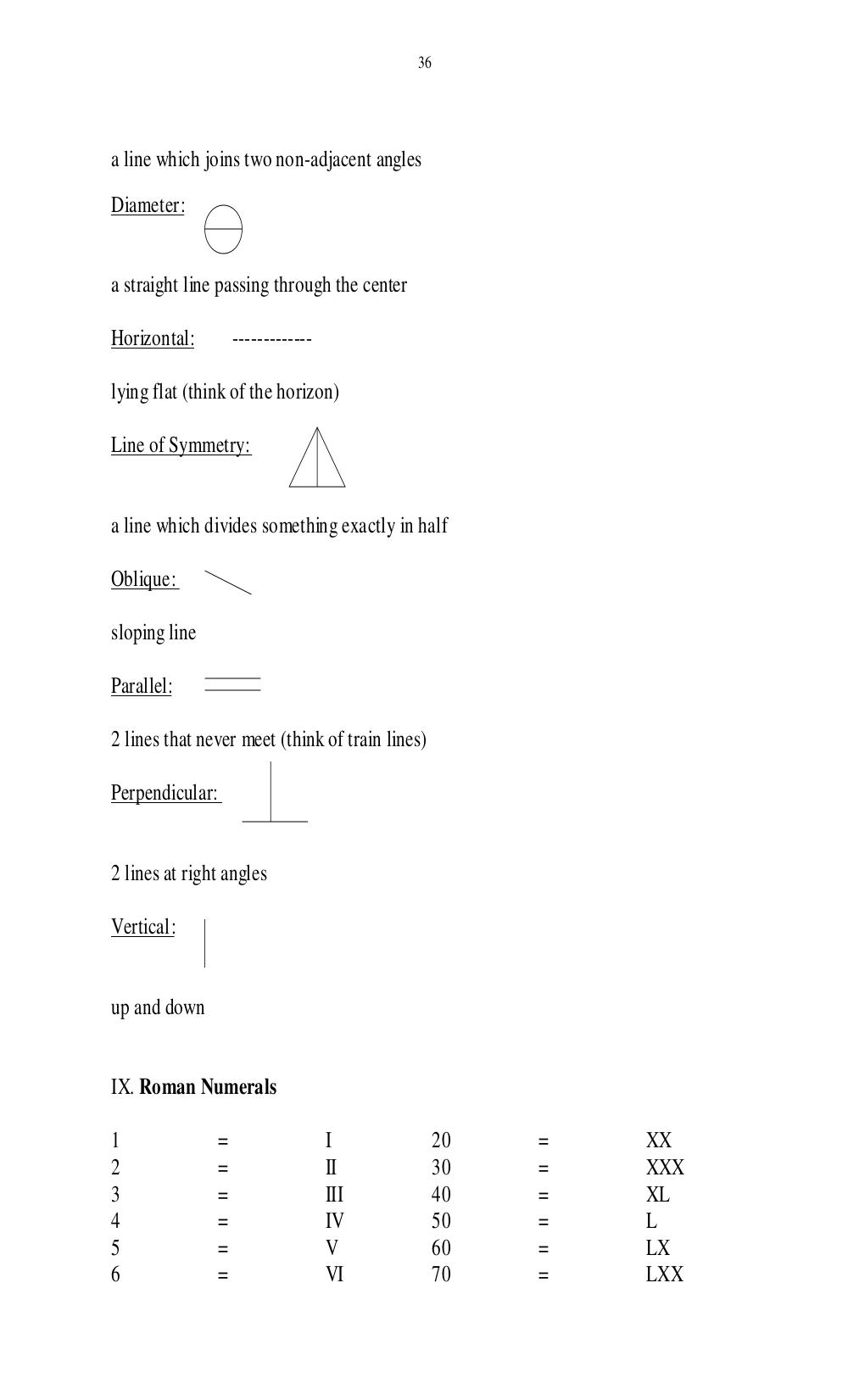# Учебно-методическое пособие по чтению специальной литературы для студентов 1 курса физического факультета. Дроздова И.В - 36 стр.

Составители:

Рубрика:

• ## Иностранный язык36
a line which joins two non-adjacent angles
Diameter:
a straight line passing through the center
Horizontal: -------------
lying flat (think of the horizon)
Line of Symmetry:
a line which divides something exactly in half
Oblique:
sloping line
Parallel:
2 lines that never meet (think of train lines)
Perpendicular:
2 lines at right angles
Vertical:
up and down
IX. Roman Numerals
1 = I 20 = XX
2 = II 30 = XXX
3 = III 40 = XL
4 = IV 50 = L
5 = V 60 = LX
6 = VI 70 = LXX36

a line which joins two non-adjacent angles

Diameter:

a straight line passing through the center

Horizontal:          -------------

lying flat (think of the horizon)

Line of Symmetry:

a line which divides something exactly in half

Oblique:

sloping line

Parallel:

2 lines that never meet (think of train lines)

Perpendicular:

2 lines at right angles

Vertical:

up and down

IX. Roman Numerals

1                =                   I            20   =   XX
2                =                   II           30   =   XXX
3                =                   III          40   =   XL
4                =                   IV           50   =   L
5                =                   V            60   =   LX
6                =                   VI           70   =   LXX